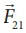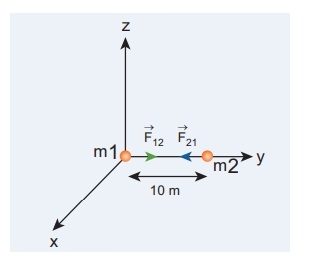Home | | Physics 11th std | Solved Example Problems for Universal Law of Gravitation

# Solved Example Problems for Universal Law of Gravitation

Physics : Gravitation - Solved Example Problems for Universal Law of Gravitation

### EXAMPLE 6.1

Consider two point masses mand m2 which are separated by a distance of 10 meter as shown in the following figure. Calculate the force of attraction between them and draw the directions of forces on each of them. Take m1= 1 kg and m2 = 2 kgSolution

The force of attraction is given byFrom the figure, r =10 m.

First, we can calculate the magnitude of the forceIt is to be noted that this force is very small. This is the reason we do not feel the gravitational force of attraction between each other. The small value of G plays a very crucial role in deciding the strength of the force.

The force of attraction () experienced by the mass m2 due to m1 is in the negative ŌĆśyŌĆÖ direction ie., r╦å =ŌłÆj╦å . According to NewtonŌĆÖs third law, the mass m2 also exerts equal and opposite force on m1. So the force of attraction () experienced by m1 due to m2 is in the direction of positive ŌĆśyŌĆÖ axis ie., r╦å = j╦å .The direction of the force is shown in the figure,Gravitational force of attraction between m1 and m2= ŌłÆwhich confirms NewtonŌĆÖs third law.

EXAMPLE 6.2

Moon and an apple are accelerated by the same gravitational force due to Earth. Compare the acceleration of the two.

The gravitational force experienced by the apple due to EarthHere MŌĆō Mass of the apple, MEŌĆō Mass of the Earth and R ŌĆō Radius of the Earth.

Equating the above equation with NewtonŌĆÖs second law,Simplifying the above equation we get,Here aA is the acceleration of apple that is equal to ŌĆśgŌĆÖ.

Similarly the force experienced by Moon due to Earth is given byHere Rm- distance of the Moon from the Earth, Mm ŌĆō Mass of the Moon

The acceleration experienced by the Moon is given byThe ratio between the appleŌĆÖs acceleration to MoonŌĆÖs acceleration is given byFrom the Hipparchrus measurement, the distance to the Moon is 60 times that of Earth radius. Rm = 60R.The appleŌĆÖs acceleration is 3600 times the acceleration of the Moon.

The same result was obtained by Newton using his gravitational formula. The appleŌĆÖs acceleration is measured easily and it is 9.8 m sŌłÆ2 . Moon orbits the Earth once in 27.3 days and by using the centripetal acceleration formula, (Refer unit 3).which is exactly what he got through his law of gravitation.

Study Material, Lecturing Notes, Assignment, Reference, Wiki description explanation, brief detail
11th Physics : UNIT 6 : Gravitation : Solved Example Problems for Universal Law of Gravitation |